# Free Falling Body Simulation Instructions on how to

• Slides: 18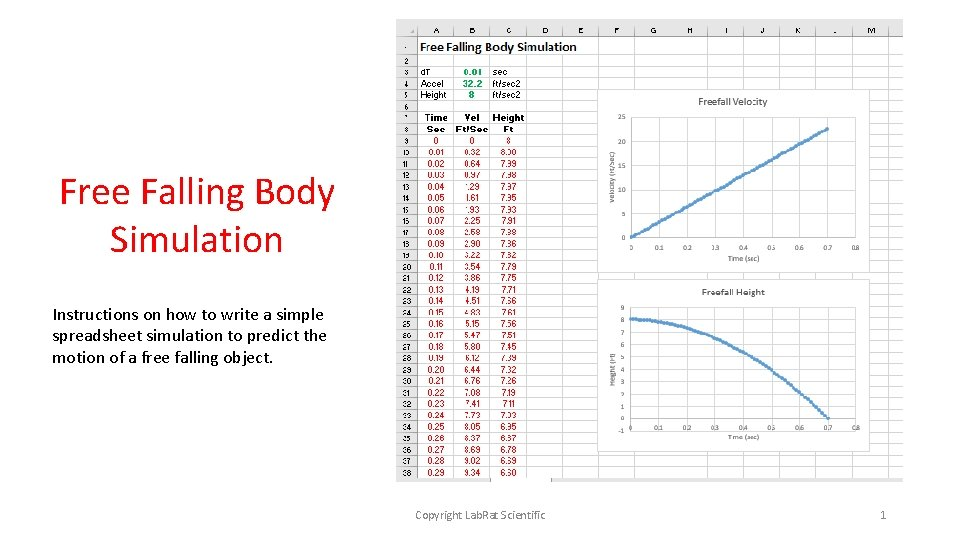Free Falling Body Simulation Instructions on how to write a simple spreadsheet simulation to predict the motion of a free falling object. Copyright Lab. Rat Scientific 1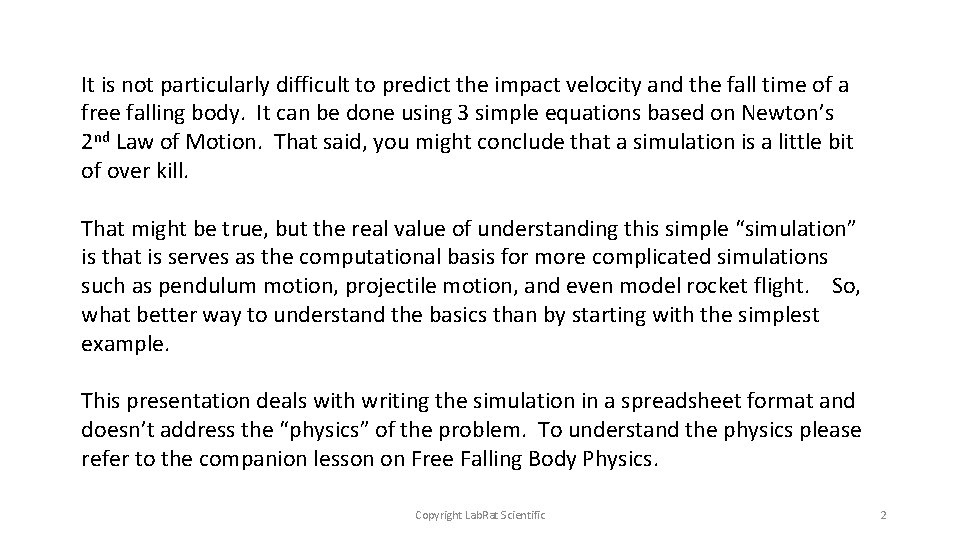It is not particularly difficult to predict the impact velocity and the fall time of a free falling body. It can be done using 3 simple equations based on Newton’s 2 nd Law of Motion. That said, you might conclude that a simulation is a little bit of over kill. That might be true, but the real value of understanding this simple “simulation” is that is serves as the computational basis for more complicated simulations such as pendulum motion, projectile motion, and even model rocket flight. So, what better way to understand the basics than by starting with the simplest example. This presentation deals with writing the simulation in a spreadsheet format and doesn’t address the “physics” of the problem. To understand the physics please refer to the companion lesson on Free Falling Body Physics. Copyright Lab. Rat Scientific 2Input Table As with all simulations, the first step is to create a table of input data. The physics and computational approach behind this simulation requires only 3 inputs. The “d. T” is the integration time step. In this case, a calculation is made for every 0. 01 sec of motion. The “Accel” is the acceleration due to gravity. This simulation is based on English units. 32. 2 ft/sec 2 represents Earth, but you could use moon gravity, Mars gravity, and Saturn gravity, etc. The “Height” is simply the height from which the object is dropped. The simulation ends when the object’s height becomes zero (i. e. hits the ground) Copyright Lab. Rat Scientific NOTE: GREEN numbers denote values that can be changed by the user. RED test is calculated data. These visual cues help prevent overwriting equations… You could also “lock” cells to prevent messing things up… 3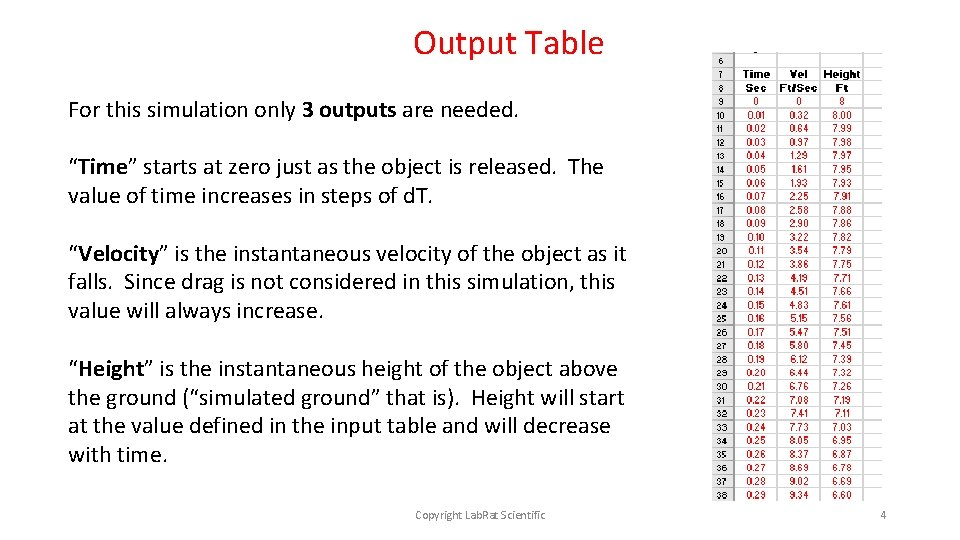Output Table For this simulation only 3 outputs are needed. “Time” starts at zero just as the object is released. The value of time increases in steps of d. T. “Velocity” is the instantaneous velocity of the object as it falls. Since drag is not considered in this simulation, this value will always increase. “Height” is the instantaneous height of the object above the ground (“simulated ground” that is). Height will start at the value defined in the input table and will decrease with time. Copyright Lab. Rat Scientific 4One of the problems with using a spreadsheet for making a simulation is that it doesn’t know when to “stop”. If you don’t include enough computational rows, the height data will never reach zero (the typical stopping point). If you have too many computational rows, the height will eventually become negative. This is not a problem with the computational approach, its just an artifact of using a spreadsheet instead of using some sort of programming language such as C++. Either delete the rows with negative data of just ignore it. When plotting the data, adjust the data set to only include heights above zero. Copyright Lab. Rat Scientific 5Data Plots The best way to examine the data is to plot it – its much easier to see if something strange is going on with the simulation. Again, this simulation isn’t very complicated so plots aren’t critical, but as the simulations become more complex, plots are critical to assessing the information. Copyright Lab. Rat Scientific 6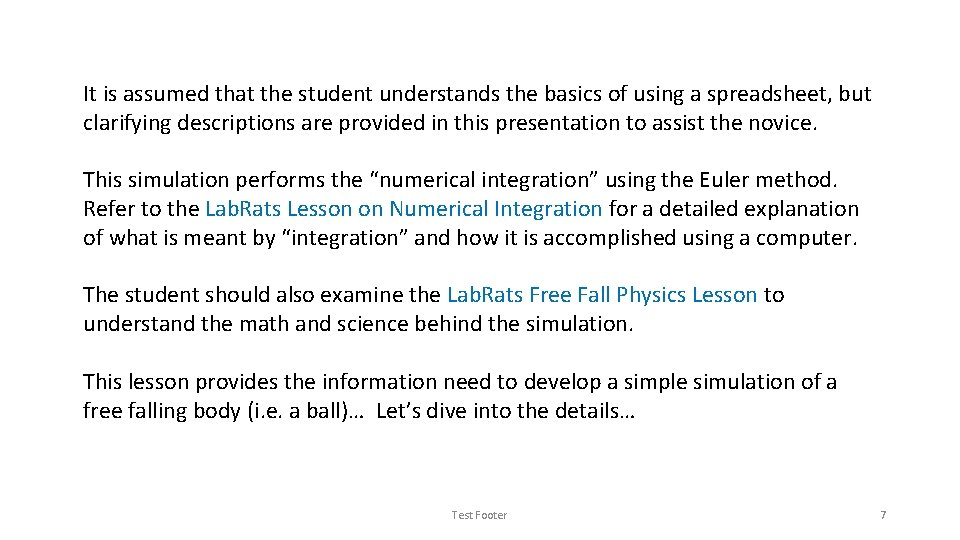It is assumed that the student understands the basics of using a spreadsheet, but clarifying descriptions are provided in this presentation to assist the novice. This simulation performs the “numerical integration” using the Euler method. Refer to the Lab. Rats Lesson on Numerical Integration for a detailed explanation of what is meant by “integration” and how it is accomplished using a computer. The student should also examine the Lab. Rats Free Fall Physics Lesson to understand the math and science behind the simulation. This lesson provides the information need to develop a simple simulation of a free falling body (i. e. a ball)… Let’s dive into the details… Test Footer 7The following slides identify the equations that are used in the simulations. The format used to convey this information is as follows: Designates the COLUMN where the info is to be entered B 10 = (A 2 * 100) / (C 7) Designates the ROW where the info is to be entered The text or equations in the cell NOTE: Make sure you enter the information of equations on the cells designated. If you don’t, the simulations won’t work since the equations reference specific cells… Copyright Lab. Rat Scientific 8Let’s look at the first ROW of the output table. The output table is actually where all of the spreadsheet calculations are made. Row 9 (First line of the computations): This represents Time = 0 (the time just when the object is dropped). This line is generally mostly zeros or an echo of some of the input data (i. e. Height). 9 A 0 B 0 C = B 5 Recall from the input table that B 5 is the starting height… Copyright Lab. Rat Scientific 9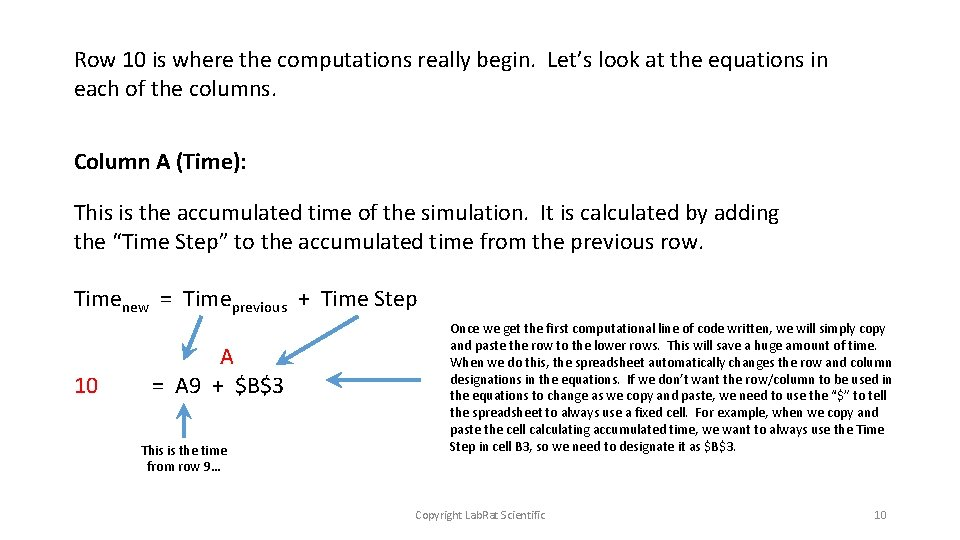Row 10 is where the computations really begin. Let’s look at the equations in each of the columns. Column A (Time): This is the accumulated time of the simulation. It is calculated by adding the “Time Step” to the accumulated time from the previous row. Timenew = Timeprevious + Time Step 10 A = A 9 + \$B\$3 This is the time from row 9… Once we get the first computational line of code written, we will simply copy and paste the row to the lower rows. This will save a huge amount of time. When we do this, the spreadsheet automatically changes the row and column designations in the equations. If we don’t want the row/column to be used in the equations to change as we copy and paste, we need to use the “\$” to tell the spreadsheet to always use a fixed cell. For example, when we copy and paste the cell calculating accumulated time, we want to always use the Time Step in cell B 3, so we need to designate it as \$B\$3. Copyright Lab. Rat Scientific 10Before we move on to the next column, it might be good to review some basic free-falling physics. When drag is neglected, a body falls at a constant acceleration. In the case of this simulation we are assuming 32. 2 Ft/Sec 2. This simulation uses a simple Euler numerical integration scheme to calculate the velocity and distance based on the instantaneous acceleration of the body. In the case of the free falling body, the instantaneous acceleration is a constant 32. 2. Since it is constant, it doesn’t make a whole lot of sense to display it for every time step. However, in more complex simulations, instantaneous acceleration changes over time and it should be displayed. Now on to calculating the velocity and height of the falling object… Copyright Lab. Rat Scientific 11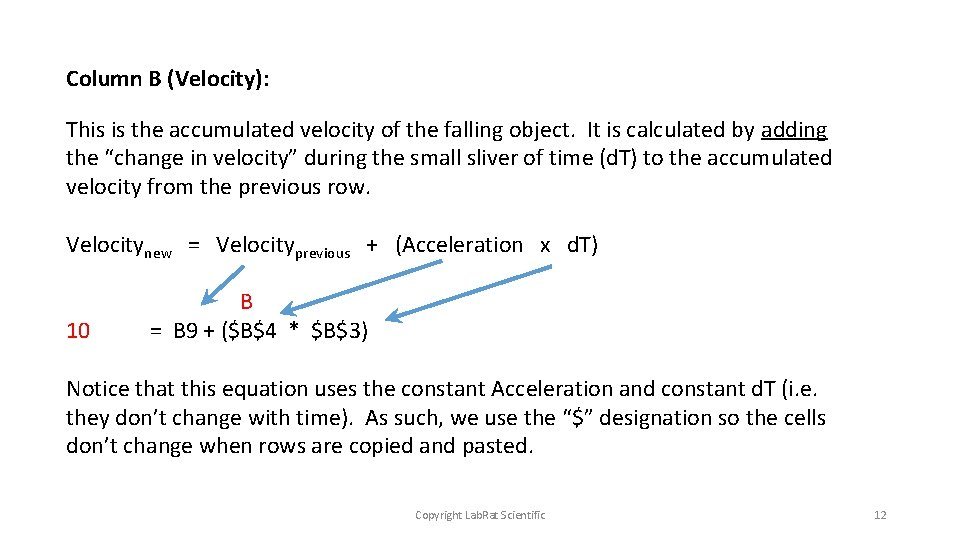Column B (Velocity): This is the accumulated velocity of the falling object. It is calculated by adding the “change in velocity” during the small sliver of time (d. T) to the accumulated velocity from the previous row. Velocitynew = Velocityprevious + (Acceleration x d. T) 10 B = B 9 + (\$B\$4 * \$B\$3) Notice that this equation uses the constant Acceleration and constant d. T (i. e. they don’t change with time). As such, we use the “\$” designation so the cells don’t change when rows are copied and pasted. Copyright Lab. Rat Scientific 12Column C (Height): This is the accumulated height of the falling object. It is calculated by subtracting the distance the object has fallen during the small sliver of time (d. T) from the height in the previous row. Heightnew = Heightprevious - (Velocity x d. T) 10 C = C 9 + (B 9 * \$B\$3) In this cell, the velocity does change with time, and thus we need to use a value from the previous row (B 9). As such, we don’t use \$B\$9… However, we are still using the “fixed” time step d. T, so we use \$B\$3 so the same cell is used even when we cut and paste subsequent rows. Copyright Lab. Rat Scientific 13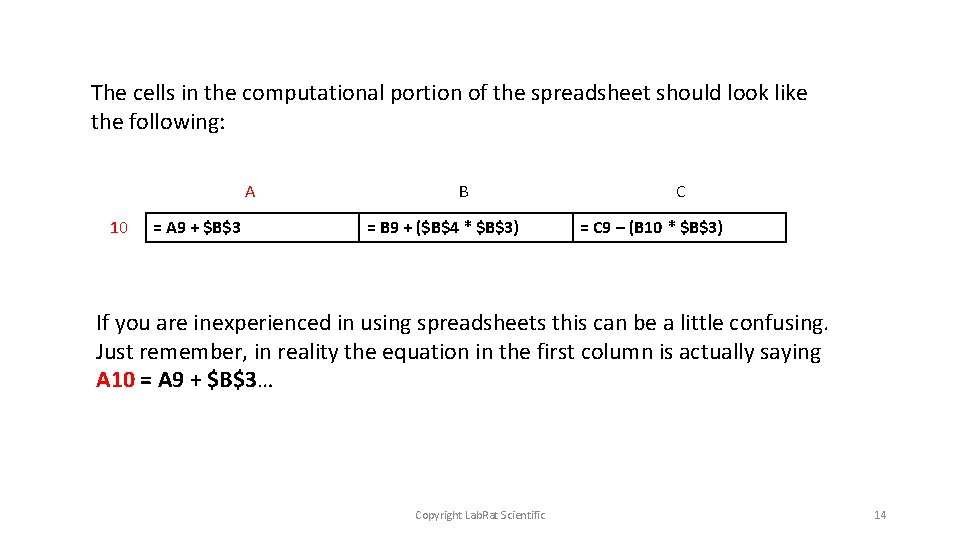The cells in the computational portion of the spreadsheet should look like the following: A 10 = A 9 + \$B\$3 B = B 9 + (\$B\$4 * \$B\$3) C = C 9 – (B 10 * \$B\$3) If you are inexperienced in using spreadsheets this can be a little confusing. Just remember, in reality the equation in the first column is actually saying A 10 = A 9 + \$B\$3… Copyright Lab. Rat Scientific 14Now its just a matter of copying ROW 10 into ROW 11, then into ROW 12 and so on. This can be done in one fail swoop. 1. Highlight an area 3 columns wide (Columns A, B & C in this case) in Row 10 by putting the cursor on Column A, then left click and hold, then move the cursor over to Column C 2. Then right click and select “Copy” 3. Move down to Row 11 and highlight the same 3 columns, but then drag the cursor down to highlight another 80 rows. 4. Then right click and select “Paste” 5. You should notice that those 80 rows display data… If you did everything correctly. You should notice that the height is near ZERO on the last row of data (depending on how many rows you actually copied. ) If not, you may have done something wrong… Copyright Lab. Rat Scientific 15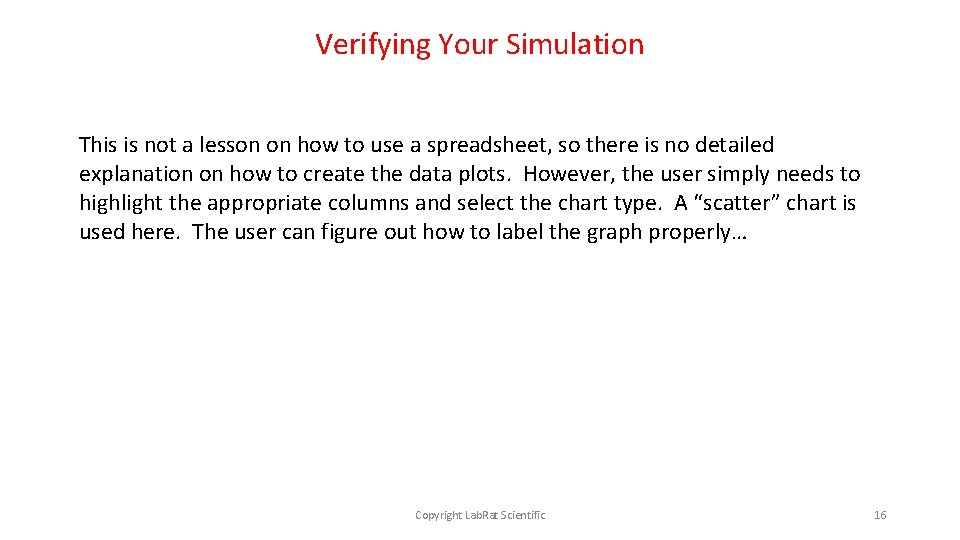Verifying Your Simulation This is not a lesson on how to use a spreadsheet, so there is no detailed explanation on how to create the data plots. However, the user simply needs to highlight the appropriate columns and select the chart type. A “scatter” chart is used here. The user can figure out how to label the graph properly… Copyright Lab. Rat Scientific 16Verifying Your Simulation This is the fun part… Before you can use your simulation to predict the behavior of a free falling object, you need to make sure it is working correctly. The best way to do this is to conduct an experiment. Use a stopwatch (or your phone) to measure the time is take a ball hit the ground when dropped from a height of 8 feet (or whatever the initial height is in your simulation). Since you may not start and stop the clock exactly at release or impact, conduct 5 or 10 drops and take an average of the results. Is your simulation matching the experiment? If not, you need to look for bugs in your spreadsheet. Copyright Lab. Rat Scientific 17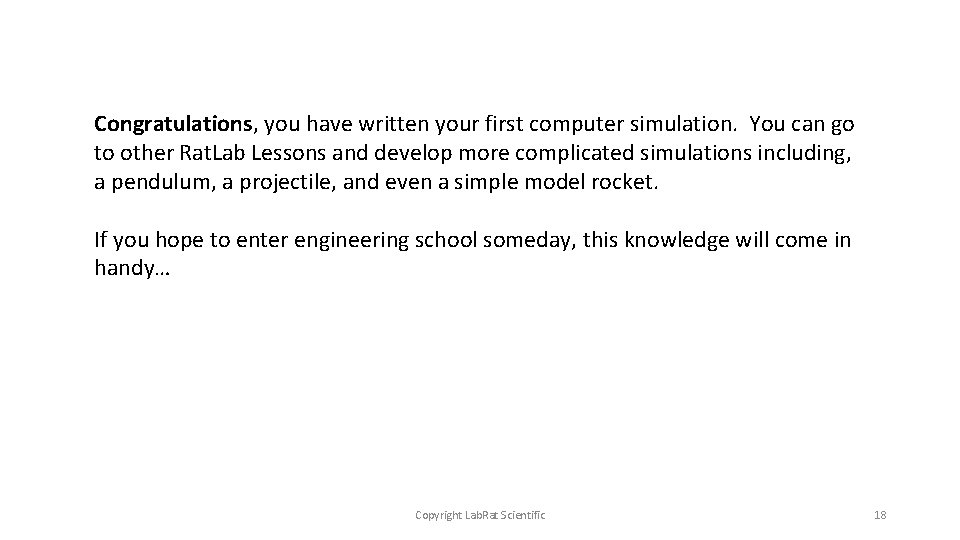Congratulations, you have written your first computer simulation. You can go to other Rat. Lab Lessons and develop more complicated simulations including, a pendulum, a projectile, and even a simple model rocket. If you hope to enter engineering school someday, this knowledge will come in handy… Copyright Lab. Rat Scientific 18